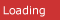# C# CornerRelated resources for python functions
• Multiple Linear Regression11/11/2019 3:43:29 AM. This is the fifteenth article in the series. Multiple linear regression (MLR), also known simply as multiple regression, is a statistical technique that uses several explanatory variables to predict t
• Logistic Regression11/10/2019 8:51:05 AM. This is the fourteenth tutorial in the series. Logistic regression is a statistical model that in its basic form uses a logistic function to model a binary dependent variable, although many more compl
• Linear Regression10/30/2019 2:32:26 AM. This is the thirteenth tutorial in the series. In statistics, linear regression is a linear approach to modeling the relationship between a scalar response and one or more explanatory variables. The c
• Data Science- A Beginner's Tutorial10/28/2019 5:55:48 AM. This is the twelfth tutorial in this series. Data science is a multi-disciplinary field that uses scientific methods, processes, algorithms, and systems to extract knowledge and insights from structur
• All about Deep Learning Tutorial10/23/2019 4:33:04 AM. This is the eleventh tutorial in the series. In this tutorial, we will be studying Deep Learning. Deep learning is a subset of machine learning in artificial intelligence (AI) that has networks capabl
• All about Machine Learning10/22/2019 1:03:23 AM. This is the tenth tutorial in the series. In this tutorial, we will be studying Machine Learning. Machine learning is the scientific study of algorithms and statistical models that computer systems us
• Artificial Intelligence - a Beginner's Tutorial10/21/2019 4:43:34 AM. This is the ninth tutorial in the series. In this tutorial, we will be studying Artificial Intelligence and its functionalities. Artificial intelligence (AI) is the simulation of human intelligence pr
• Python Basics10/19/2019 4:52:17 AM. Python is a scripting language, which is an open-source language. It finds its use as one of the most powerful machine learning languages. Here, we will take an overview of Python language
• A Complete Python Seaborn Tutorial10/17/2019 5:21:44 AM. This is the seventh tutorial in the series. In this tutorial, we will be studying about seaborn and its functionalities. Seaborn is a Python data visualization library based on matplotlib. It provides
• A Complete Python TensorFlow Tutorial10/17/2019 5:20:16 AM. This is the eighth tutorial in the series. In this tutorial, we will be studying about Tensorflow and its functionalities. TensorFlow is a free and open-source software library for dataflow and differ
• Python Functions Overview10/24/2015 8:59:50 AM. In this article I will explain you how to create and call functions in python.